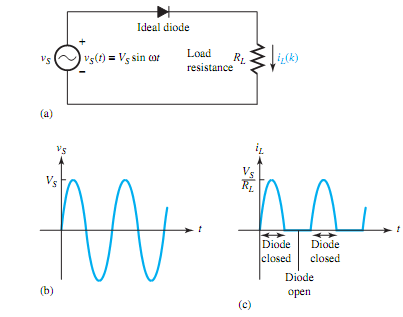## Explain the working of rectifier circuits, Electrical Engineering

Assignment Help:

Q. Explain the working of Rectifier Circuits?

A simple half-wave rectifier using an ideal diode is shown in Figure(a). The sinusoidal source voltage vS is shown in Figure (b). During the positive half-cycle of the source, the ideal diode is forward-biased and closed so that the source voltage is directly connected across the load. During the negative half-cycle of the source, the ideal diode is reverse-biased so that the source voltage is disconnected from the load and the load voltage as well as the load current are zero. The load voltage and current are of one polarity and hence said to be rectified. The output current through the load resistance is shown in Figure (c).In order to smooth out the pulsations (i.e., to eliminate the higher frequency harmonics) of the rectified current, a filter capacitor may be placed across the load resistor, as shown in Figure (a). As the source voltage initially increases positively, the diode is forward-biased since the load voltage is zero and the source is directly connected across the load. Once the source reaches its maximum value VS and begins to decrease, while the load voltage and the capacitor voltage are momentarily maintained at VS, the diode becomes reverse-biased and hence open- circuited. The capacitor then discharges over time interval t2 through RL until the source voltage vS(t) has increased to a value equal to the load voltage. Since the source voltage at this point in time exceeds the capacitor voltage, the diode becomes once again forward-biased and hence closed. The capacitor once again gets charged to VS. The output current of the rectifier with the filter capacitor is shown in Figure(b), and the circuit configurations while the capacitor gets charged and discharged are shown in Figure(c). The smoothing effect of the filter can be improved by increasing the time constant CRL so that the discharge rate is slowed and the output current more closely resembles a true dc current.

#### Related Discussions:- Explain the working of rectifier circuits

Disadvantages of SDR 1. Power consumption is more due to rapid switching of modes 2. Hardware design is not easy 3. Software bugs exist in the system

#### Motor: power production, The mechanical power produced at the armature of a...

The mechanical power produced at the armature of a motor is: P = T a . (power is in watts if torque is N.m and    in rad/sec). Some of this will be lost due to friction and

#### Explain thevenin''s theorem procedure, Thevenin's theorem procedure:   1. ...

Thevenin's theorem procedure:   1.  Open circuit R L and find Thevenin's voltage (V TH ). 2.  Find Thevenin's resistance (R TH ) when voltage source is short circuit or curren

#### Explain polling, What is meant by polling? In polling schemes, a set of...

What is meant by polling? In polling schemes, a set of address lines is driven by the controller to address every of the masters in sequence. When a bus request is received fro

#### Voltmeter transistor, explain basic working of voltmeter transistor

explain basic working of voltmeter transistor

#### Calculate the pay back of the project, Problem: Jyoti Textile is consid...

Problem: Jyoti Textile is considering whether to add a new product to its range. Machinery costing \$280,000 would have to be bought at the start of the project (Year 0). The pr

#### For zero flag , For zero flag  CZ ( Call  on Zero )  CNZ ( Call on no...

For zero flag  CZ ( Call  on Zero )  CNZ ( Call on no zero ) Instructions CZ call the  subroutine  from the  specified  memory location if zero flag is set  (Z=1). The i

#### Self inductance , If a current is passed through a coil, it creates a flux ...

If a current is passed through a coil, it creates a flux within the coil. Any attempt to change this flux will create a back emf that acts to oppose the change.   Consider again

#### Comparison of analog-modulation systems, Comparison of Analog-Modulation Sy...

Comparison of Analog-Modulation Systems The comparison of analog modulation systems (conventional AM, DSB SC, SSB SC, VSB, FM, and PM) can be based on three practical criteria:

#### Nop no operation instruction , NOP No Operation Instruction No operati...

NOP No Operation Instruction No operation  is performed  when this  instruction is executed. The instruction format is             NOP All  registers and flags  re